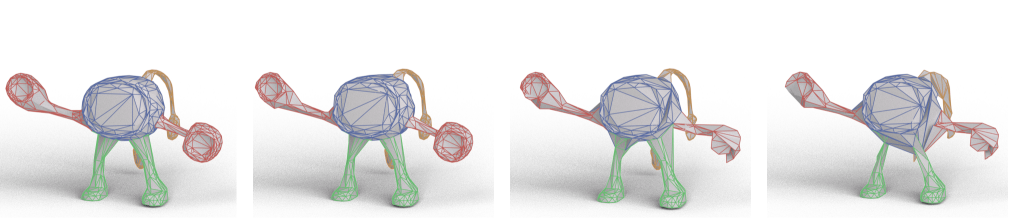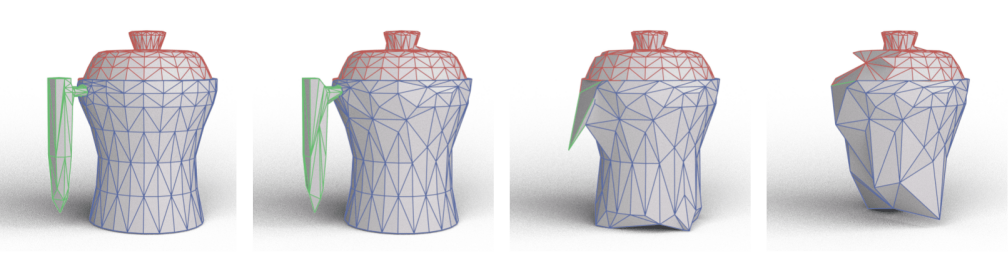# MeshCNN: A Network with an Edge

Rana Hanocka1   Amir Hertz1   Noa Fish1   Raja Giryes1   Shachar Fleishman2   Daniel Cohen-Or1
1Tel Aviv University     2Amazon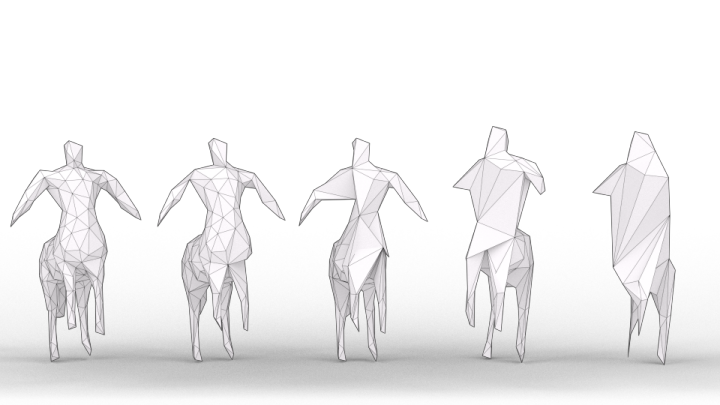## Abstract

Polygonal meshes provide an efficient representation for 3D shapes. They explicitly capture both shape surface and topology, and leverage non-uniformity to represent large flat regions as well as sharp, intricate features. This non-uniformity and irregularity, however, inhibits mesh analysis efforts using neural networks that combine convolution and pooling operations. In this paper, we utilize the unique properties of the mesh for a direct analysis of 3D shapes using MeshCNN, a convolutional neural network designed specifically for triangular meshes. Analogous to classic CNNs, MeshCNN combines specialized convolution and pooling layers that operate on the mesh edges, by leveraging their intrinsic geodesic connections. Convolutions are applied on edges and the four edges of their incident triangles, and pooling is applied via an edge collapse operation that retains surface topology, thereby, generating new mesh connectivity for the subsequent convolutions. MeshCNN learns which edges to collapse, thus forming a task-driven process where the network exposes and expands the important features while discarding the redundant ones. We demonstrate the effectiveness of our task-driven pooling on various learning tasks applied to 3D meshes.

## The Layers of MeshCNN

In MeshCNN the edges of a mesh are analogous to pixels in an image, since they are the basic building blocks for all CNN operations. Just as images start with a basic input feature: an RGB value per pixel; MeshCNN starts with a few basic geometric features per edge. The input edge feature is a 5-dimensional vector every edge: the dihedral angle, two inner angles and two edge-length ratios for each face.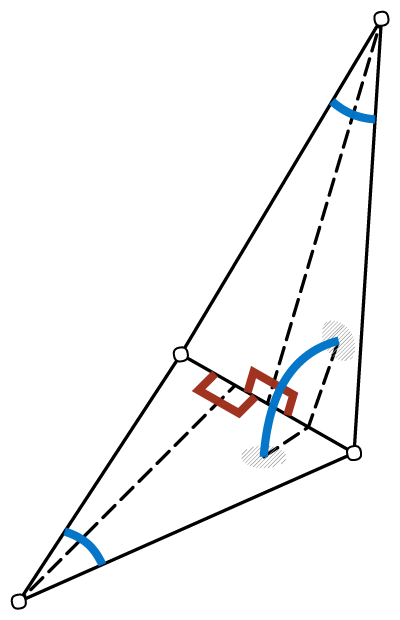#### Input Edge Features

MeshCNN learns features on the edges of the mesh, since every edge is incident to exactly two faces (triangles), which defines a natural fixed-sized convolutional neighborhood of four edges.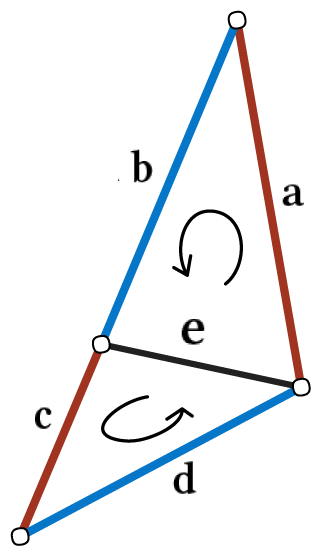#### Mesh Convolution

Learned convolutional filters are applied on each edge feature vector and the 4 one-ring neighbors. The consistent face normal order is used to apply a symmetric convolution operation, which learns edge features that are invariant to rotations, translations and uniform scale. Mesh pooling downsamples the number of features in the network, by performing a edge-collapse on the learned edge features. The new edge neighbors are computed dynamically inside the network, and used in the next convolutions.#### Mesh Pooling & Unpooling

For fully-convolutional tasks (such as segmentation), a mesh unpooling operation is used to restore the original mesh resolution.

## Results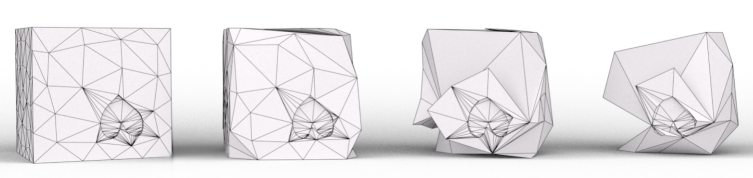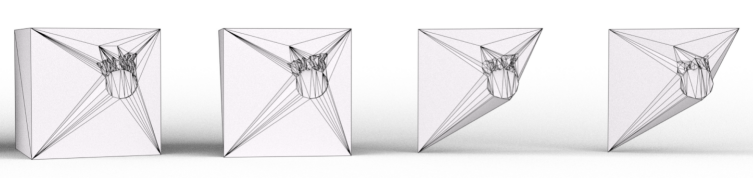#### Learned Simplifications on Cube Dataset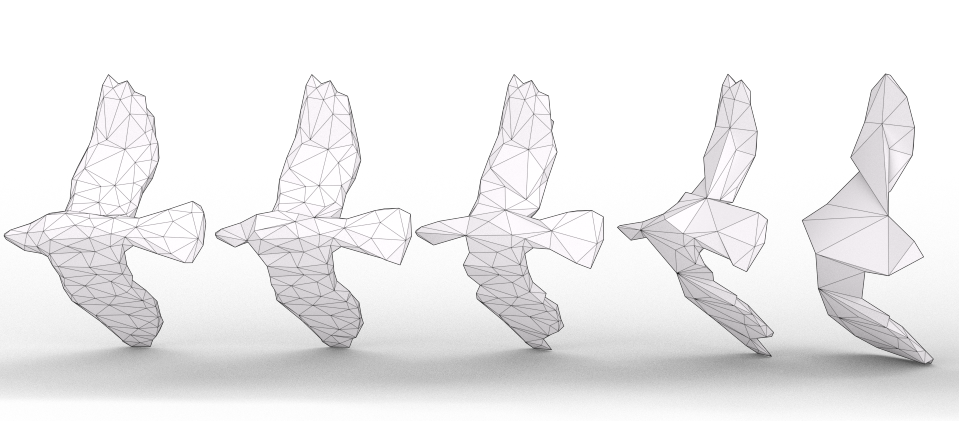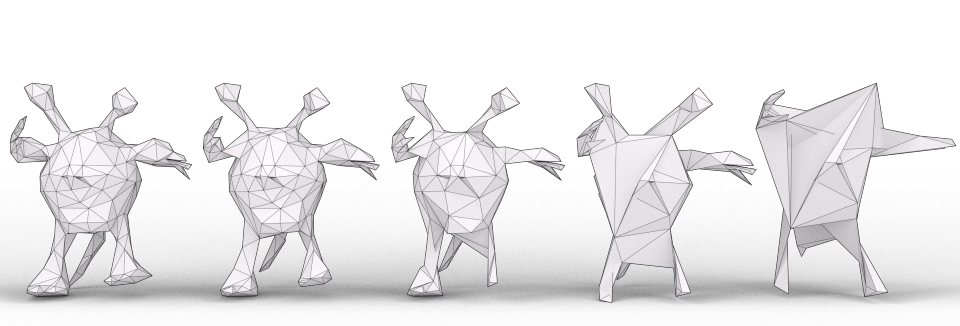#### Learned Simplifications on Shrec Dataset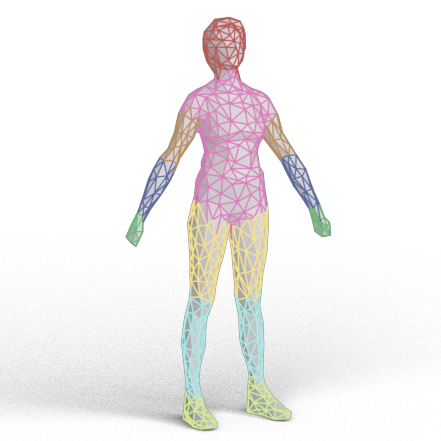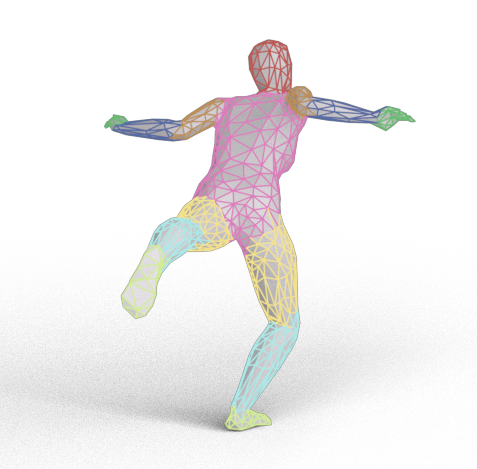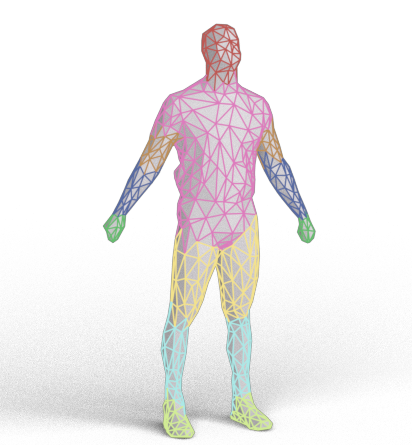#### Human Segmentation Results